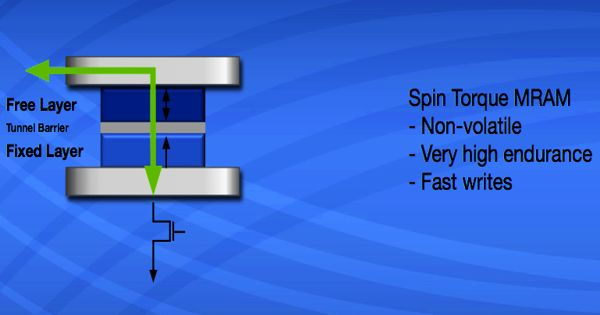Physics

# Spin-transfer torqueSpin-transfer torque (STT) is an effect in which the orientation of a magnetic layer in a magnetic tunnel junction or spin valve can be modified using a spin-polarized current. Spin transfer torque occurs in situations where a current of spin-polarized carriers from the left part of a device with a particular polarization (given by the unit vector S1) enters the right part of the device with a different magnetization direction (given by the unit vector S2).

Charge carriers (such as electrons) have a property known as spin which is a small quantity of angular momentum intrinsic to the carrier. When the electrons incoming from the left side enter the right magnetic part, they will eventually be polarized along S2, meaning that the right magnetic domain has exerted a torque on the electrons in order to rotate their spin angular momentum.

An electric current is generally unpolarized (consisting of 50% spin-up and 50% spin-down electrons); a spin-polarized current is one with more electrons of either spin. However, due to the conservation of angular momentum, the electrons exert an equal but opposite torque on the right magnetic domain – the spin-transfer torque. By passing a current through a thick magnetic layer (usually called the “fixed layer”), one can produce a spin-polarized current. Consider an electric current through some magnetically polarized material, it will in general exhibit some finite spin polarization. If this spin-polarized current is directed into a second, thinner magnetic layer (the “free layer”), the angular momentum can be transferred to this layer, changing its orientation. If this current flows through an area where the state of magnetization corresponds to a different orientation of the spin polarization, then somehow the carriers have to adapt to this.

The spin-transfer torque may cause either a magnetization precession in the ferromagnetic electrode or a reversal of the magnetization of the electrode. The spin-transfer torque is utilized as a recording mechanism in the spin-transfer-torque magnetic random access memory (STT-MRAM).

The spin-transfer torque can be applied to the controllable switching of two magnetic layers in a recording nanopillar bit in MRAM. This can be used to excite oscillations or even flip the orientation of the magnet. The effects are usually seen only in nanometer-scale devices. Two important issues have to be solved for the immediate application of the spin-transfer switching in MRAM.

Information Source: